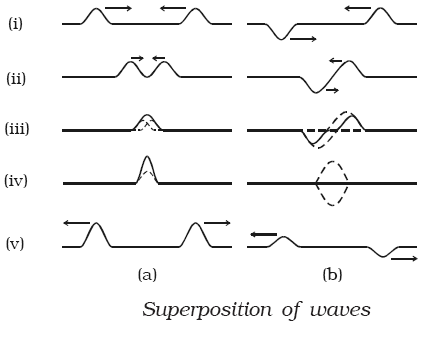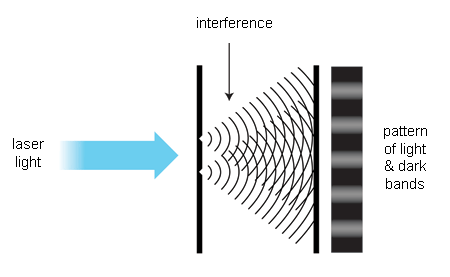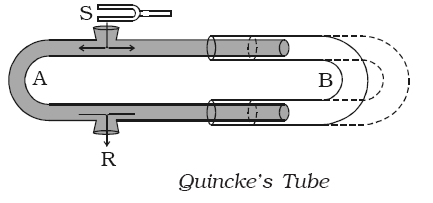Click to Chat

1800-1023-196

+91-120-4616500

CART 0

• 0

MY CART (5)

Use Coupon: CART20 and get 20% off on all online Study Material

ITEM
DETAILS
MRP
DISCOUNT
FINAL PRICE
Total Price: Rs.

There are no items in this cart.
Continue Shopping• Complete Physics Course - Class 11
• OFFERED PRICE: Rs. 2,968
• View Details

```Interference of Sound Wave

Superposition principle

When two waves travel in a medium simultaneously in such a way that each wave represents its separate motion, then the resultant displacement at any point at any time is equal to the vector sum of the individual displacements of the waves.This principle is illustrated by means of a slinky as shown in the figure.

(a) In the figure, (i) shows that the two pulses pass each other,

(b) In the figure, (ii) shows that they are at some distance apart

(c) In the figure, (iii) shows that they overlap partly

(d) In the figure, (iv) shows that  resultant is maximum

Figure illustrates the (ii) same events but with pulses that are equal and opposite. (iii)

If Y1   and Y2 are the (iv) displacements at a point, then the resultant displacement is (v)

given by Y = Y1 +Y2 .

If |Y1 |=|Y2 |= a,  and if the two waves have their displacements in the same direction, then |Y| = a + a = 2a

If the two waves have their displacements in the opposite direction, then |Y | = a + (-a) = 0

The principle of superposition of waves is applied in wave phenomena such as interference, beats and stationary waves.

Interference of wavesWhen two waves of same frequency travelling in the same direction in a medium superpose with each other, their resultant intensity is maximum at some points and minimum at some other points. This phenomenon of superposition is called interference.

Let us consider two simple harmonic waves of same frequency travelling in the same direction. If a1 and a2 are the amplitudes of the waves and φ is the phase difference between them, then their instantaneous displacements are

y1 = a1sin ωt          …... (1)

y2 = a2sin (ωt + φ)     …... (2)

According to the principle of superposition, the resultant displacement is represented by

y = y1 + y2

= a1 sin ωt + a2 sin (ωt + φ)

= a1 sin ωt + a2 (sin ωt. cos φ + cos ωt.sin φ)

= (a1 + a2 cos φ) sin ωt + a2 sin φ cos ωt           …... (3)

Put a1 + a2 cos φ = A cos θ               …... (4)

a2 sin φ = A sin θ                             …... (5)

where A and θ are constants, then

y = A sin ωt. cos θ + A cos ωt. sin θ

or  y = A sin (ωt + θ)

This equation gives the resultant displacement with amplitude A. From eqn. (4) and (5),

This equation gives the resultant displacement with amplitude A. From equation (4) and (5),

A2cos2θ + A2sin2θ = (a1+a2cosφ)2 + (a2sinφ)2

So, A2 = a12 + a22 + 2a1a2cosφ

Thus, A = √[a12 + a22 + 2a1a2cosφ]           …... (7)

Also tanθ = a2 sinφ/[a1+a2 cosφ]               …... (8)

We know that intensity is directly proportional to the square of the amplitude

So, I ∝ A2

Thus, I ∝ (a12 + a22 + 2a1a2 cosφ)           …... (9)

Special cases

The resultant amplitude A is maximum, when cosφ = 1 or φ = 2mπ where m is an integer (i.e) Imax ∝ (a1+a2)2

The resultant amplitude A is minimum when

cosφ = -1 or φ = (2m + 1)π

Imin ∝ (a1 – a2)2

The points at which interfering waves meet in the same phase φ = 2mπ i.e 0, 2π, 4π, ... are points of maximum intensity, where constructive interference takes place. The points at which two interfering waves meet out of phase φ = (2m + 1)π i.e π, 3π, ... are called points of minimum intensity, where destructive interference takes place.

Refer this video to know more about interference of wave

Experimental demonstration of interference of soundThe phenomenon of interference between two longitudinal waves in air can be demonstrated by Quincke’s tube shown in figure. Quincke’s tube consists of U shaped glass tubes A and B. The tube SAR has two openings at S and R. The other tube B can slide over the tube A. A sound wave from S travels along both the  paths  SAR  and  SBR  in opposite  directions  and  meet at R.

If the path difference between the two waves (i.e) SAR ~ SBR is an integral multiple of wavelength, intensity of sound will be maximum due to constructive interference.

i.e  SAR ~ SBR = mλ

The corresponding phase difference φ between the two waves is even multiples of π. (i.e) φ = m 2π where m = 0, 1, 2, 3 ....

If the tube B is gradually slided over A, a stage is reached when the intensity of sound is zero at R due to destructive interference. Then no sound will be heard at R.

If the path difference between the waves is odd multiples of λ/2 , intensity of sound will be minimum.

i.e  SAR ~ SBR = (2m + 1) λ/2

The corresponding phase difference φ between the two waves is odd multiples of π. (i.e) φ = (2m + 1)π where m = 0, 1, 2, 3 .....When two waves interfere, the resulting displacement of the medium at any location is the algebraic sum of the displacements of the individual waves at that same location.

The disturbances of waves are superimposed when they come together—a phenomenon called superposition.

As a result of superposition of waves, interference can be observed. Interference is an effect caused by two or more waves. Waves can interfere constructively or destructively.

The superposition of most waves produces a combination of constructive and destructive interference and can vary from place to place and time to time.

The two sources must emit continuous waves having same frequency and wave length.

The amplitudes of two waves must either be equal or nearly equal.

The two sources should be situated close to each other.

The two sources should be coherent one.

Problem (JEE Main):

In a plane progressive harmonic wave particle speed is always less than the wave speed if

(a) amplitude of wave is less than  λ/2π.

(b) amplitude of wave is greater than  λ/2π.

(c) amplitude of wave is less than λ.

(d) amplitude of wave is greater than  λ/π.

(λ = wavelength)

Solution:

Let equation of a plane progressive harmonic wave be,

y = A sin (ωt – kx)

wave speed, v = ω/k

and maximum particle speed, vp = Aω

Given that, vp < v

Or, Aω < ω/k

Or, A < 1/k

Or, A< λ/2π

Thus, from the above observation we conclude that, option (a) is correct.Question 1

Which of the following statements is correct:

(a) both sound and light waves in air are longitudinal.

(b) both sound and light waves in air are transverse.

(c) sound waves in air are transverse and light waves are longitudinal.

(d) sound waves in air are longitudinal and light waves are transverse.

Question 2

The reciprocal of wavelength is called_____

a) frequency

b) wave velocity

c) wave number

d) amplitude

Question 3

When the particles of a medium are vibrating at right angles to the direction of energy transport, then the wave is a ____ wave.

(a) transverse       (b) sound

(c) standing           (d) longitudinal

Question 4

Many wave properties are dependent upon other wave properties. Yet, one wave property is independent of all other wave properties. Which one of the following properties of a wave is independent of all the others?

(a) wavelength          (b) frequency

(c) period                  (d) velocity

Question 5

If the frequency of a wave is doubled and if the speed remains constant, its wavelength is ____.

(a) quartered             (b) halved

(c) unchanged           (d) doubledQ.1
Q.2
Q.3
Q.4
Q.5

d

c

a

d

b

Related Resources:-

You might like to refer progressive wave.

For getting an idea of the type of questions asked, refer the  Previous Year Question Papers.

To read more, Buy study material of Waves comprising study notes, revision notes, video lectures, previous year solved questions etc. Also browse for more study materials on Physics here.
```### Course Features

• 101 Video Lectures
• Revision Notes
• Previous Year Papers
• Mind Map
• Study Planner
• NCERT Solutions
• Discussion Forum
• Test paper with Video Solution